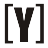# Electrical Units

## Electrical Units table

 Name Symbol Quantity Ampere (amp) A Electric current (I) Volt V Voltage (V, E) Electromotive force (E) Potential difference (Δφ) Ohm Ω Resistance (R) Watt W Electric power (P) Decibel-milliwatt dBm Electric power (P) Decibel-Watt dBW Electric power (P) Volt-Ampere-Reactive var Reactive power (Q) Volt-Ampere VA Apparent power (S) Farad F Capacitance (C) Henry H Inductance (L) siemens / mho S Conductance (G) Admittance (Y) Coulomb C Electric charge (Q) Ampere-hour Ah Electric charge (Q) Joule J Energy (E) Kilowatt-hour kWh Energy (E) Electron-volt eV Energy (E) Ohm-meter Ω∙m Resistivity (ρ) siemens per meter S/m Conductivity (σ) Volts per meter V/m Electric field (E) Newtons per coulomb N/C Electric field (E) Volt-meter V⋅m Electric flux (Φe) Tesla T Magnetic field (B) Gauss G Magnetic field (B) Weber Wb Magnetic flux (Φm) Hertz Hz Frequency (f) Seconds s Time (t) Meter / metre m Length (l) Square-meter m2 Area (A) Decibel dB Parts per million ppm

### Units Prefix table

Prefix Symbol Factor Example
pico p 10-12 1pF = 10-12F
nano n 10-9 1nF = 10-9F
micro μ 10-6 1μA = 10-6A
milli m 10-3 1mA = 10-3A
kilo k 103 1kΩ = 1000Ω
mega M 106 1MHz = 106Hz
giga G 109 1GHz = 109Hz

### Electrical Units Definitions

Ampere (A)
Electrical unit of electrical current and measures the amount of electrical charge in an electrical circuit (per 1 second).

`1A = 1C / 1s`

Volt (V)
Electrical unit of voltage.

`1V = 1J / 1C`

Ohm (Ω)
Electrical unit of resistance.

`1Ω = 1V / 1A`

Watt (W)
Electrical unit of electric power and measures the rate of consumed energy.

`1W = 1J / 1s`
`1W = 1V ⋅ 1A`

Decibel-milliwatt (dBm)
Unit of electric power, measured with logarithmic scale referenced to 1mW.

`10dBm = 10 ⋅ log10(10mW / 1mW)`

Decibel-Watt (dBW)
Unit of electric power, measured with logarithmic scale referenced to 1W.

`10dBW = 10 ⋅ log10(10W / 1W)`

Unit of capacitance. It represents the amount of electric charge in coulombs that is stored per 1 volt.

`1F = 1C / 1V`

Henry (H)
Unit of inductance.

`1H = 1Wb / 1A`

siemens (S)
Unit of conductance, which is the opposite of resistance.

`1S = 1 / 1Ω`

Coulomb (C)
Unit of electric charge.

`1C = 6.238792×1018 electron charges`

Ampere-hour (Ah)
Ampere-hour is a unit of electric charge.

One ampere-hour

`1Ah = 1A ⋅ 1hour`
`1Ah = 3600C`

Tesla (T)
Unit of magnetic field.

`1T = 1Wb / 1m2`

Weber (Wb)
Unit of magnetic flux.

`1Wb = 1V ⋅ 1s`

Joule (J)
Unit of energy.

`1J = 1 kg ⋅ m2 / s2`

Kilowatt-hour (kWh)
Unit of energy.

`1kWh = 1kW ⋅ 1h = 1000W ⋅ 1h`

Kilovolt-amps (kVA)
Unit of power.

`1kVA = 1kV ⋅ 1A = 1000 ⋅ 1V ⋅ 1A`

Hertz (Hz)
Unit of frequency. It measures the number of cycles per second.

`1 Hz = 1 cycles / s`YoosFuhl.com Would you like to receive notifications on latest updates? No Yes